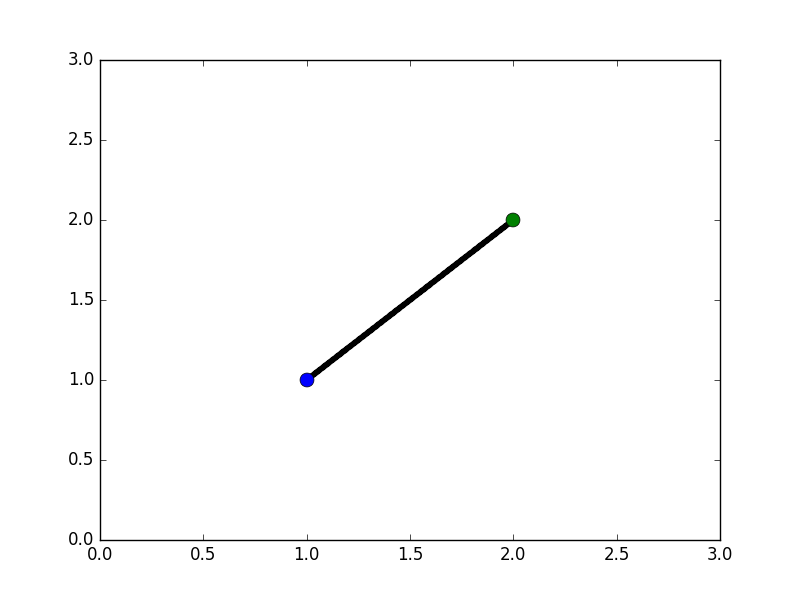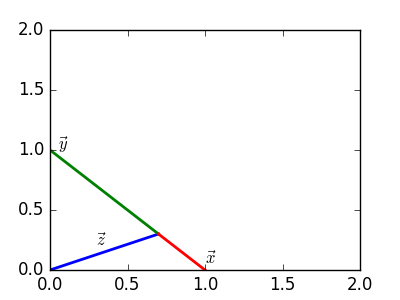• 页面上有两颗星星图标，画一条线连接两颗星星。 1.先获取两点的坐标 2.计算弦长及旋转角度 3.绘制线 <div class="quality-analysis"> <div class="cnt-box"> <span class="selected-start"> ...
页面上有两颗星星图标，画一条线连接两颗星星。

1.先获取两点的坐标   2.计算弦长及旋转角度   3.绘制线

<div class="quality-analysis">
<div class="cnt-box">
<span  class="selected-start">
<svg class="icon fs16 warn-text icon-orange " aria-hidden="true">
</svg>
</span>
<span  class="selected-start">
<svg class="icon fs16 gray-text" aria-hidden="true">
</svg>
</span>
</div>
</div>

<script>
export default {
data() {
return {
positionList: [], // 获取坐标
}
},
mounted() {
this.getStartPosition()
this.drawLine(this.positionList)
},
methods: {
// 获取星星坐标
getStartPosition() {
let nodeList = document.querySelectorAll('.selected-start')
let parentNode = document.querySelector('.cnt-box').offsetParent
let actualLeft = 0
let current = 0
let actualTop = 0
if(nodeList.length>0){
for(let i=0;i<nodeList.length;i++){
actualLeft = nodeList[i].offsetLeft  // 累加计算星星相对于顶级父元素的距离
actualTop = nodeList[i].offsetTop
current = nodeList[i].offsetParent
while(current!== parentNode){
actualLeft += current.offsetLeft
actualTop += current.offsetTop
current = current.offsetParent
}
let obj = {
x:actualLeft,
y:actualTop,
}
this.positionList.push(obj)
}
}

},

// 画星星连线
drawLine(positionList) {
if(positionList.length>0){
for(let i=1;i<positionList.length;i++){
// 计算长宽
let rectWidth = Math.abs(positionList[i].x - positionList[i-1].x)
let rectHeight = Math.abs(positionList[i].y - positionList[i-1].y)
// 在页面确定div左上角的具体位置
let rectX = positionList[i].x < positionList[i-1].x ? positionList[i].x : positionList[i-1].x
let rectY = positionList[i].y < positionList[i-1].y  ? positionList[i].y : positionList[i-1].y

// 弦长
let stringWidth = Math.ceil(Math.sqrt((rectWidth * rectWidth)+ (rectHeight*rectHeight)))
let xPad = (rectWidth - stringWidth)/2
let yPad = (rectHeight - 2)/2
// 从x轴到(x,y)的旋转角度
let radNum = Math.atan2((positionList[i].y - positionList[i-1].y),(positionList[i].x - positionList[i-1].x))
let  traX  = rectX +  xPad - 8 // 8为星星图标大小的1/2
let  traY  = rectY +  yPad + 8
// 创建div
let div = document.createElement('div')
div.innerHTML = '<div ' +
' style = "width:' + stringWidth + 'px;height:2px;transform:translate(' + traX+ 'px,' + traY +'px) rotate('+ radNum+ 'rad) " class="draw-line-style"></div>'
let doc = document.querySelector('.quality-analysis')
doc.appendChild(div)
}
}
}
}
}
</script>

说明：

<style lang="scss">
.quality-analysis {
position: relative;
.draw-line-style{
top: 0;
position: absolute;
border-top: 1px solid \$bgOrange;
}
</style>

1.每个元素都有offsetTop和offsetLeft属性，表示该元素的左上角与父容器（offsetParent对象）左上角的距离。所以，只需要将这两个值进行累加，就可以得到该元素的绝对坐标。

2.atan2(y,x) ：返回从 x 轴到点 (x,y) 的角度（介于 -PI/2 与 PI/2 弧度之间）。

展开全文• 个不同A,B确定一条直线，AB相同返回的值全0 直线方程：Ax+By+c=0 A = y2 - y1; B = x1 - x2; C = -Ax1 - By1 = x2y1 - x1y2; 证明之后补上； Line LineMake(Point A, Point B) { Line l; l.A = B.y - A.y; l.B...
两个不同点A,B确定一条直线，AB相同返回的值全0

直线方程：Ax+By+c=0
A = y2 - y1;
B = x1 - x2;
C = -Ax1 - By1 = x2y1 - x1y2;

证明之后补上；
Line LineMake(Point A, Point B)
{
Line l;
l.A = B.y - A.y;
l.B = A.x - B.x;
l.C = B.x * A.y - A.x * B.y;
return l;
}


展开全文• 如何由两点坐标确定一条直线的ax+by+c=0表达式 比如已知两坐标： (x1,y1),(x2,y2); 对于表达式ax+by+c=0; a=?,b=?,c=?; y=kx+m, y1=kx1+m y2=kx2+m, k=(y2-y1)/(x2-x1) m=y1-(y2-y1)x1/(x2-x1) y=(y2-y1)/(x2-x1)x+...
如何由两点坐标确定一条直线的ax+by+c=0表达式
比如已知两坐标：
(x1,y1),(x2,y2);
对于表达式ax+by+c=0;
a=?,b=?,c=?;
y=kx+m,
y1=kx1+m
y2=kx2+m,
k=(y2-y1)/(x2-x1)
m=y1-(y2-y1)x1/(x2-x1)
y=(y2-y1)/(x2-x1)x+(y1(x2-x1)-x1(y2-y1))/(x2-x1)
(y1-y2)x+(x2-x1)y+(x1y2-x2y1)=0,
a=y1-y2,
b=x2-x1,
1
c=x1y2-x2y1
2
a=a/gcd(a,b);
b=b/gcd(a,b)
c=-ax-by;
借鉴于：https://www.zybang.com/question/6413f293470b3cbb7e2b0ca6d89c4da3.html


展开全文• 计算机能画一条线段（数学意义上）吗？...已知 x,yx,y 分别为坐标轴上的两点，下式为采样公式， x′=(1−p)⋅x+p⋅y,p∈[0,1] x'=(1-p)\cdot x+p\cdot y,\quad p\in [0, 1] 对 pp在整个 [0,1][0, 1]闭区间上
计算机能画一条线段（数学意义上）吗？并不能。不管多短的一条线段，都包含实数集上的无数个点，计算机能做的是尽可能多地采样，描更多的点，让人眼难以看出其中的不连续性。

已知 x,y<!--//--><![CDATA[//><!--
x,y
//--><!]]> 分别为坐标轴上的两点，下式为采样公式， x′=(1−p)⋅x+p⋅y,p∈[0,1]<!--//--><![CDATA[//><!--
x'=(1-p)\cdot x+p\cdot y,\quad p\in [0, 1]
//--><!]]>对 p<!--//--><![CDATA[//><!--
p
//--><!]]>在整个 [0,1]<!--//--><![CDATA[//><!--
[0, 1]
//--><!]]>闭区间上取值，可得到介于端点 x,y<!--//--><![CDATA[//><!--
x,y
//--><!]]> 之间的值，因为向量 (x′−x)=p⋅(y−x)<!--//--><![CDATA[//><!--
(x'-x) =p\cdot (y-x)
//--><!]]> 平行于向量 y−x<!--//--><![CDATA[//><!--
y-x
//--><!]]>，又因为二者共享同一端点 x<!--//--><![CDATA[//><!--
x
//--><!]]>，故三者共线。

import numpy as np
import matplotlib.pyplot as plt

def main():
x0, x1 = [1, 1], [2, 2]
for p in np.linspace(0, 1, 1000):
plt.plot((1-p)*x0+p*x1, (1-p)*x0+p*x1, '.', c='k')
plt.plot(x0, x0, 'o', ms=10)
plt.plot(x1, x1, 'o', ms=10)
plt.show()
if __name__ == '__main__':
main()我们不妨对该公式做一次简单推导，如图所示：x⃗ −z⃗ <!--//--><![CDATA[//><!--
\vec x-\vec z
//--><!]]> 与 z⃗ −y⃗ <!--//--><![CDATA[//><!--
\vec z-\vec y
//--><!]]> 共线，不妨令  x⃗ −z⃗ =k(z⃗ −y⃗ )⇓x⃗ +ky⃗ =(1+k)z⃗ ⇓z⃗ =11+kx⃗ +k1+ky⃗ ⇓11+k→λ,11+k≤1z⃗ =λx⃗ +(1−λ)y⃗ <!--//--><![CDATA[//><!--
\vec x-\vec z=k(\vec z-\vec y)\\
\Downarrow\\
\vec x+k\vec y=(1+k)\vec z\\
\Downarrow\\
\vec z=\frac1{1+k}\vec x+\frac{k}{1+k}\vec y\\
\Downarrow_{\frac1{1+k}\rightarrow \lambda,\;\frac1{1+k}\leq1}\\
\vec z=\lambda\vec x+(1-\lambda)\vec y
//--><!]]>
展开全文• /* 个不同A,B确定一条直线，AB相同返回的值全0 * 直线方程：Ax+By+c=0 * A = y2 - y1; * B = x1 - x2; * C = -A*x1 - B*y1 = x2*y1 - x1*y2; */ Line LineMake(Point A, Point B) { Line l; l.A...语言 c
• 如上图，已知四个(x0,y0)、(x1,y1)、(x2,y2)、(x3,y3)，其中(x0,y0)、(x1,y1)确定直线1，(x2,y2)、(x3,y3)确定直线2，求直线1、直线2的交点(x,y)，公式如下： y = ( (y0-y1)*(y3-y2)*x0 + (y3-y2)*(x1-x0)*y0 + ...
• 平面上N个，每确定一条直线， 求出斜率最大的那条直线所通过的（斜率不存在的情况不考虑）。时间效率越高越好。 平面上N个，每确定一条直线，求出斜率最大的那条直线所通过的...
• 问题： 平面上N个，每之间可以确定一条直线，求出最大斜率。时间效率越高越好。 方法： 1. 先把N个按x排序。2. 斜率k最大值为max(斜率(point[i],point[i+1])) 0 <=i <n-2。 3. 复杂度Nlog(N) ...
• 平面上N个，没确定一条直线，求出斜率最大的那条直线所通过的（斜率不存在的情况不考虑）。时间效率越高越好。先把N个按x排序。 斜率k最大值为max(斜率(point[i],point[i+1])) 0 复杂度Nlog(N)。...
• 确定逆向地理编码时服务商对地址的方位没有清楚的描述，导致偏远的地区没有明确的描述，此算法通过个坐标的相对位置计算出了方位角得到方位，可以清楚的描述 A地址距离B地址南北方向5000米，类似这样的说明。...
• （3）两条垂线的交点就是圆心 2.计算圆的半径 根据图，实际是求三角形OBC的斜边OB长度。C是AB的中点 （1）A，B已知，角a也就已知了。并且CB=AB/2 （2）CB/OB=sin(c) =&gt;OB= CB/sin(c) （3）...
• 种方法都是求一条最短路径，如果你想求多条最短路径那就只能选择其他方法了。 网上已经有几种算法可以求多条最短路径，最常见的就是删边法+di。就是用狄克斯特拉求出一条最短路径然后把最短路径上的边一条一条的...Floyd
• 按照数据结构课本上的说法： 前序遍历+中序遍历 后序遍历+中序遍历 可以唯一确定一棵二叉树。 反例： 1 1 / \ 1 1 上述棵二叉树的前序序列和中序序列都为（1，1）...
• ...如图已知:a、b两点的坐标, c到a的距离d 。求c点的坐标。 求 cy 可以根据d / a到b距离 = a到c垂直距离 / a 到 b的垂直距离。 首先求a到b的距离 r = sqrt((x1- x2)*(x1 - x2) +...坐标
• 判断最多有多少个能再一条直线上（n 解析：求任意构成的直线的方程 化为一般式:ax+by+c=0 得： a=y2-y1 b=x1-x2//注意别写反了 c=-ax1+by1 即可 不可思议的n = 700时n ^3也能过 #include算法 计算几何
• 如何根据空间中两点确定一条曲线 绘制的电线可以用鼠标点击 最终实现的效果如下: 现在我们来一一解决。 贝塞尔曲线 关于贝塞尔曲线的原理，讲解的文章很多，大多是列出公式，然后就提供代码，其实讲得大同小异，...曲线 Unity3D
• 首先我们来看一下投影分类。 从上图中我们可以看出来透视投影是平面几何投影的大类。其下又有一点透视、二点透视和三点透视之分。 我们再来看看透视投影的性质：...下面我将证明两点： 两平行直线不平行于投影...透视投影
• ## 根据两点经纬度坐标计算距离

万次阅读 多人点赞 2015-05-09 11:23:29
问题提出目前手头的个项目要用到GPS地理定位信息，很自然的就需要知道个地点之间的距离，于是上网找了一下。背景知识这些经纬线是怎样定出来的呢？地球是在不停地绕地轴旋转（地轴是根通过地球南北两极和地球...定位
• ## 遍历寻找给定两点之间的所有路径

万次阅读 多人点赞 2017-04-09 11:20:24
直接入题，参加2017年华为竞赛的时候，需要输出路径，当时设了源点和汇点，是单源单汇的寻路径，那么问题就归为寻找给定两点之间的所有路径问题 我们的老师一直教我们这些菜鸟们说，写程序一定要先在纸上写好考虑...华为 路径搜索 遍历
• 个曲线确定的交线的切向量求解@(微积分)有个很有启发性的说法：考虑描述曲面的隐函数F(x,y,z)=0.F(x,y,z)=0. 其全微分dF=F′xdx+F′ydy+F′zdz=0dF=F'_xdx+F'_ydy+F'_zdz=0,即(F′x,F′y,F′z)(dx,dy,dz)=0(F...
• 2、遍历出所有取出两点的情况(不考虑先后顺序)，根据任意两点确定一条直线，直线参数为k斜率，b与y轴交点的纵坐标（此时x=0），将他们放入一个列表中 3、将所有直线放入一个集合并完成去重操作，增加直线的第三个...
• 设已知的一条线段的起点和终点为(x1,y1),(x2,y2)，另一条为 (x3,y3),(x4,y4)；首先求出条线段的一次函数，再联立求出交点坐标交点公式： x=(y3x4x2-y4x3x2-y3x4x1+y4x3x1-y1x2x4+y2x1x4+y1x2x3-y2x1x3)/x4y2-x4y1...算法
• 半连通的定义，有向图G(V,E)，任意个不同的u、v，u有一条路径可达v或者v有一条路径可达u，从定义中可以看出，强连通图一定是半连通的。 引理：有向无环图G(V,E)，G是半连通的当且仅当有一条路径，这条路径...
• 题目描述 给你一个 n 个，m 条边的无向图，求至少要在...接下来的m行中，每行个正整数 i 、 j ，表示i与j之间有一条无向道路。 输出描述: 输出一个整数，表示答案 示例1 输入 4 2 1 2 3 4 输出 1
• 一点透视只有个灭点，通过组斜线来表示空间纵深感。下面我们将要学习的是两点透视，它通过两个灭点来增加斜线，两个面都用斜线绘制，从而增加纵深感。...什么两点透视 两点透视，也叫做透视变换 图像处理
• 1.维搜索问题维线搜索，就是指单变量函数的最优化，它是专门针对单峰函数设计的：如上篇文章所述，多变量函数中，迭代格式为：xk+1=xk+αkdkx_{k+1} = x_k + \alpha_k d_k其中的关键就在于找到合适的步长αk,最优化理论与算法
• 怎样用画笔画光滑曲线-两点三次埃尔米特插值法（转自http://blog.sina.com.cn/s/blog_a8192bdd010105rx.html） 求几点（3点以上）之间的光滑曲线是有很多办法的，如果需要曲线通过目标点，可以用经典的三次样条...
• 已知两点A(x1,y1)，B(x2,y2)，则画AB线段程序为： plot([x1,x2],[y1,y2]); 或 line([x1,x2],[y1,y2]);
• 根据两点经纬度计算距离 这些经纬线是怎样定出来的呢？地球是在不停地绕地轴旋转（地轴是根通过地球南北两极和地球中心的 假想线），在地球中腰画个与地轴垂直的大圆圈，使圈上的每一点都和南北两极的距离......